# Basic of Drillpipe Tensile Capacity and Its Calculation

This article demonstrates basic knowledge understanding of tensile capacity of the drill pipe and some calculation. First of all we need to know basic of material strength and for our case is strength of metal.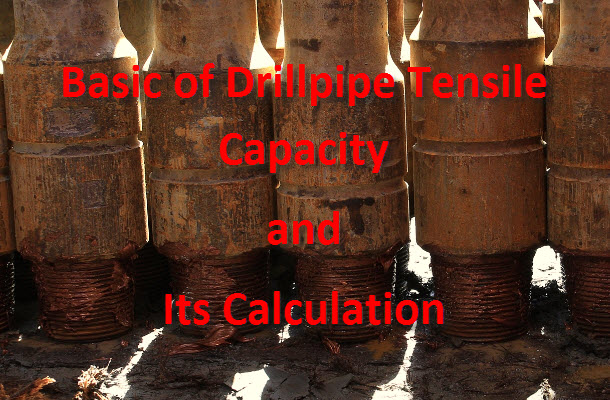There are few simple terminologies which we would like to explain.

## Stress (σ)

Stress (σ) equals to force divided by cross sectional area of the material (F/A). For our case, we will discuss about only stress in tensile because the drill pipe is almost always designed to work in a tensile condition.

### Stress (σ) = F/A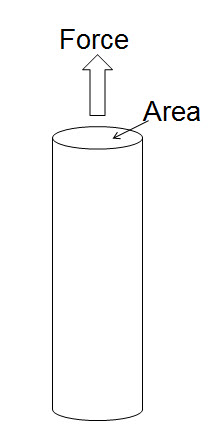Figure 1 – Stress (σ) = F/A

## Strain (ε)

Strain (ε) is a change of material per an original length. From the definition, it equals to ∆L/L (see Figure 2).

### Strain (ε) = ∆L/LFigure 2 – Strain Relationship

## Young’s Modulus (Modulus of Elasticity)

Young’s modulus (the tensile modulus or elastic modulus) is a ratio of stress and strain along the axis and we can write into the following equation.

### Young’s modulus = Stress (σ) ÷ Strain (ε) = (F x L) ÷ (∆L x A)

Where;

F is pulling force.

L is an original length of pipe.

∆L is an amount by which the length of the pipe changes.

A is a cross sectional area of object.

The Young’s Modulus of material represents the factor of proportional in Hook’s Law therefore it will valid under the elastic zone.  There are several units for Young’s Modulus as N/m2 (Newton), Maga Pascal (N/mm2) and Pound per Square Inch (psi).

## Stress-Strain Curve

A stress-strain curve is a graph derived from Stress (σ) versus Strain (ε) for a sample of a material. The nature of the curve varies from material to material. The following curve shows a behavior of metal.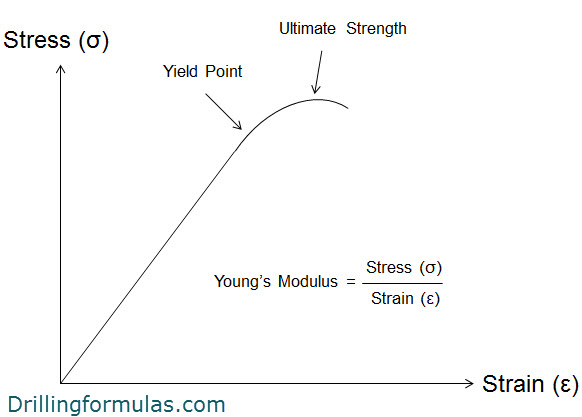Figure 3 – Stress-Strain Curve

Yield Point or Yield strength, is defined as the stress at which a material begins to plastically deform. Before the yield point the material will deform elastically and it will return to its original shape when the stress is released. If the tension applied is over the yield point, the deformation will be permanent and non-reversible.

Ultimate strength is the maximum stress applied before the material is completely parted.

Young’s Modulus (modulus of elasticity) is the slope of the Stress-Strain curve within the elastic limit (see Figure 4). It means that once tensile is less than Yield Point, the Young’s Modulus is valid for the calculation.

Young’s Modulus of steel is 30 x 106 psi.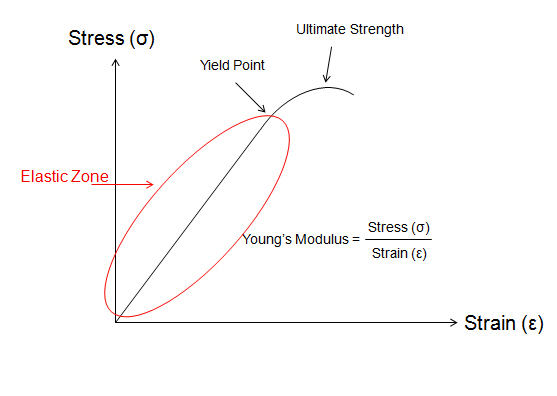Figure 4– Young’s Modulus in The Elastic Zone

In drilling operation, we must operate within Yield point because the metal will become the original shape. For example, if you get stuck, the maximum tension applied to free the stuck drillstring must be always under yield point with a designed safety factor for the operation.

API RP7G classifies a grade of drill pipe body according to yield strength and tensile requirement (see Table 1 and Table 2). Four grades of drill pipe are “E”, “X”, “G” and “S”.

Table 1 – API Drill Pipe Grade US customary unit (Ref: API Specification 5DP Specification for Drill Pipe EFFECTIVE DATE: AUGUST 1, 2010 Page 87))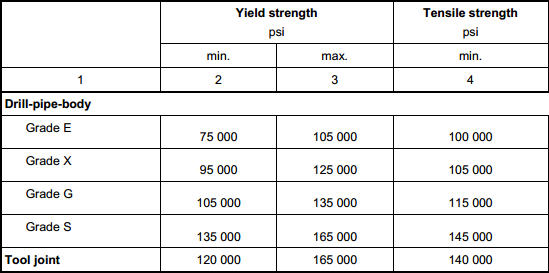Table 2 – API Drill Pipe Grade SI unit (Ref: API Specification 5DP Specification for Drill Pipe EFFECTIVE DATE: AUGUST 1, 2010 Page 55)## Drillpipe Tensile Capacity

Tensile capacity of drill pipe is maximum tension applied before the elastic limit is reached and the formula is below;

### Tensile Capacity = Cross Sectional Area x Yield Strength

In this article, we refer to US customary unit therefore the units for calculations are as follows;

Tensile Capacity is in lb.

Cross Sectional Area is in square inch.

Yield Strength is in psi.

Example

API 5”, S-135, NC50, Class New (100% Wall Thickness)

OD = 5 inch

Nominal ID = 4.276 inch

Minimum yield strength = 135,000 psi

What is the tensile capacity of this new pipe (100% wall thickness)?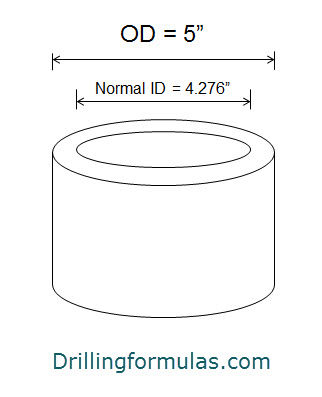Figure 5 – Diagram of new pipe (100% wall thickness)

Wall thickness = (5-4.276) ÷2 = 0.362 inch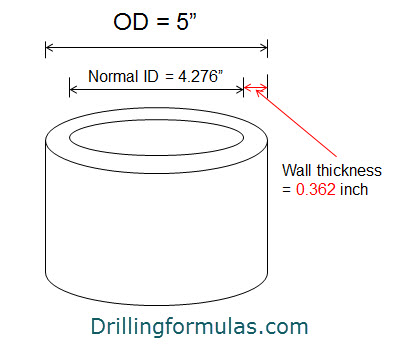Figure 6 – Wall Thickness of New Pipe

Cross Sectional Area of New Pipe = π x (OD2 – ID2) ÷ 4 = π x (5.02 – 4.2762) ÷ 4

Cross Sectional Area of New Pipe = 5.275 square inch

Tensile Capacity = Cross Sectional Area x Yield Strength

Tensile Capacity = 5.275 x 135,000 = 712,070 lb.

What is the tensile capacity of the premium class (80% wall thickness)?

Premium class is defined as the minimum of wall thickness is 80% of new pipe. We will discuss a little more about class of pipe later.

Wall thickness of new pipe = 0.362 inch

Wall thickness of premium class pipe = 0.8 x 0.362 = 0.290 inch therefore the OD is 4.856”.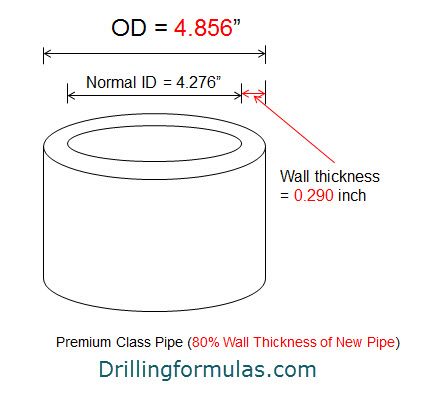Figure 7 – Wall Thickness of Premium Class Pipe

Cross Sectional Area of New Pipe = π x (OD2 – ID2) ÷ 4 = π x (4.8562 – 4.2762) ÷ 4

Cross Sectional Area of New Pipe = 4.154 square inch

Tensile Capacity = Cross Sectional Area x Yield Strength

Tensile Capacity = 4.154 x 135,000 = 560,764 lb.

As you see from the calculation, premium class drill pipe has approximately 79% of tensile of new pipe. This figure is very important for engineering design and drilling operation. For instant, if you get stuck with the premium class pipe, you must apply tension less than the tensile capacity of 560 Klb in order to ensure that you will not deform or damage your drillstring.

Please always remember that in our drilling operation, we must operate the drillstring within the tensile limit.

Share the joyWorking in the oil field and loving to share knowledge.

### 15 Responses to Basic of Drillpipe Tensile Capacity and Its Calculation

1.oyeniyi adebayo says:

sir,
i commend you on your effort to make drilling easy. please i want to read more or if there is text book for maintenance on drilling rigs and equipment

2.JAIRO C. MOLERO says:

I need to known How I calculeted for one Drill Pipe Type Class 2….Thank

3.Mian Muhammad Tahir says:

Sir,
Those you send different information regarding using of drilling is very very important for the TOOL PUSHER , RIG MANAGER & RIG LIAISON MAN persons,
Thank you & Keep it up
Rig Manager KSA

4.Alvis says:

Referring to table 1, what is the min and max yield strength and min tensile strength?

Why is there a min and max for yield strength? It’s just a point in the graph.
Is min tensile strength ultimate strength?

•DrillingFormulas.Com says:

Alvis,

There is a min and max for yield strength because the manufactures need to make the pipe within the specific window in order to meet the API requirement.

Regards,
Shyne.

5.reza says:

Dear

Thanks.
Could you please explain the difference between “min. Yield strength, max. Yield strength and min. Tensile strength” with example?

Best Regards,

•DrillingFormulas.Com says:

Min Yield strength = The stress level above which a material begins undergoing to plastic deformation.
Min Tensile strength = the minimum stress at which a material breaks.

•Hussain says:

Isn’t that just the definition of Yield point ? If material begins undergoing to plastic deformation after exceeding Min Yield strength then how is there a maximum Yield Strength ? For example, DP grade E has min YS 75k psi and max YS105k psi. if it deforms plastically after exceeding 75k psi. what is max YS ?
I would appreciate an answer, because im confused.

Thank you so much for all the valuable info.

6.reza says:

Dear Mr. Shyne

Again me!!!.
As you know the min. yield strength for all drill pipes are 120 psi. Therefore, to calculate the tensile capacity for drill pipes with min. YS more than 120 psi, for example grade S135, we should use the 120psi or not?

Regards,

•DrillingFormulas.Com says:

All standard tool joint is 120 psi but the tensile strength to meet pipe tensile strength by increase cross-sectional area. You can see that tool joint will have various size of OD and ID even though they are all 120 psi strength.

Strength = Force x Cross Sectional Area.

7.Haytham Elkamash says:

This is very useful information.
Keep up with this useful data.
Thanks.

8.omar hassan says:

thank u very much

9.Ahmed says:

thank you man
god save u for ever to us

•mohamed jamal says:

god save you guys

10.mohamed jamal says:

thanks very much

This site uses Akismet to reduce spam. Learn how your comment data is processed.